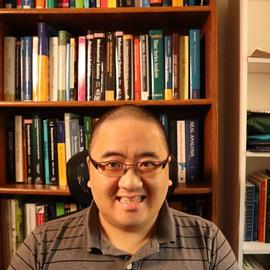# Some Proposals for Statistical Education

Views and opinions expressed are solely my own.

## Background

I hold a bachelor’s degree in mathematics (with a statistics emphasis) and a master’s degree in statistics. Statistical education, on its own, is insufficient to address modern data needs. The American Statistical Association recognized a similar sentiment in 2019 in their executive summary of the report Statistics at a Crossroads: Who Is for the Challenge?:

The field of Statistics is at a crossroads: we either flourish by embracing and leading Data Science or we decline and become irrelevant. In the long run, to thrive, we must redefine, broaden, and transform the field of Statistics. We must evolve and grow to be the transdisciplinary science that collects and extracts useful information from data. With the fast establishment of various Data Science entities across campuses, industry, and government, there is a limited time window of opportunity for a successful transformation that we must not miss. We must effect this change now by reimagining our educational programs, rethinking faculty hiring and promotion, and accelerating the cultural change that is required.

The intent of this post is to propose solutions to some of these issues.

## Proposal 1: Stop emphasizing the normal distribution.

Every introductory statistics course and mathematical statistics course (requiring calculus) I have seen at the undergraduate level spends an inordinate amount of time on the normal distribution and its properties. Having served as a teaching assistant and tutor for such classes in the past, students are often left with the impression that normal distributions are realistic models for most real-world data. This is an obvious falsehood.

What is not pointed out in statistics courses is that normal distributions are a construct of mathematical convenience not rooted in reality. For example:

• Normal distributions have an equal mean, median, and mode. Real-world populations of data should not be expected to behave this way, and reasonably do not.1
• Independent normal distributions follow nice linearity properties. One should not expect the same for real-world data.
• Normal distributions arise as a consequence of the Central Limit Theorem. I will expand on this idea later in this post.

Statistics courses should emphasize exploratory data analysis techniques and visualizations of data more often than properties of the normal distribution.

## Proposal 2: Stop emphasizing the Central Limit Theorem.

The Central Limit Theorem (CLT) is a beautiful result in probability that makes very little sense to apply in practice, but is taught and applied ad nauseam both inside and the classroom, whether indirectly or directly. In attempting to minimize the technical jargon, to summarize the CLT:

Suppose we have a random sample $$X_1, \dots, X_n$$ taken from a population with a finite mean $$\mu$$ and finite standard deviation $$\sigma$$. Then, as the sample size $$n$$ approaches infinity, if $$\overline{X}_n = \dfrac{1}{n}\sum_{i=1}^{n}X_i$$ is the sample average, the quantity $\dfrac{\overline{X}_n - \mu}{\sigma/\sqrt{n}}$ is normally distributed with mean $$0$$ and standard deviation $$1$$.

What is important to note about the above statement of the CLT is what it doesn’t tell us, more specifically that the CLT only applies as the sample size approaches infinity. Of course, it is unrealistic to expect that one can obtain an infinite sample size. In most undergraduate-level statistics classes, rules of thumb such as “$$n \geq 30$$” or “$$np \geq 5$$ and $$n(1-p) \geq 5$$” are approximations to infinity. But there is no justification that these rules of thumb are actually sufficient estimates for infinity.

Statistics courses generally could use more coverage in permutation testing, Monte Carlo simulation, and nonparametric alternatives such as bootstrapping.

## Proposal 3: Teach statistics with a programming language.

Statistics cannot be done at a reasonable scale in practice with a handheld calculator. Over the course of my statistical education, basic tools that I use on a daily basis – such as dialects of SQL – were never even mentioned in the classroom. I am of the opinion that sometime in the future, statistics courses at the undergraduate level will either (1) require a computer-science prerequisite or (2) teach basic computer science alongside the statistics. University of California, Berkeley has already figured out the second scenario with their Data 8 course, a data course in Python requiring no prerequisites.

With the way many degree programs are currently structured, statistics at the undergraduate level is the math requirement for graduation, and computer science is often not included in general education requirements, often only being required for STEM degrees. Whether a student is in a STEM field or not – especially in marketing or in the social sciences – some computer science (usually about a semester) is necessary to get them to use data tools for tasks such as day-to-day reporting at scale, or research.

## Conclusions

Statistics will “decline and become irrelevant” if the education doesn’t resemble what is done in practice. Mathematically-convenient structures, such as the normal distribution and the Central Limit Theorem, should not be given the prominence they are given in statistical education. Whether a student is studying STEM or not, statistics in practice requires fundamental computer science skills.

1. I once had someone ask me why the mean of a data set isn’t equal to the median of that same data set.↩︎##### Yeng Miller-Chang

I am a Senior Data Scientist - Global Knowledge Solutions with General Mills, Inc. Views and opinions expressed are my own.